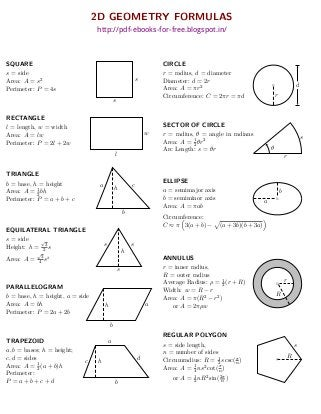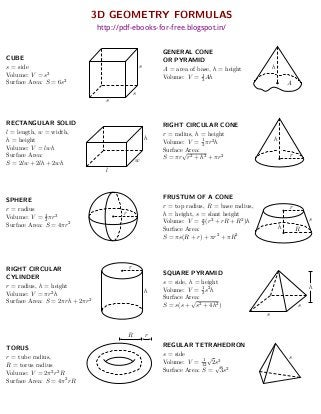Successfully reported this slideshow.

# 2D and 3D Geometry Formulas eBook×

# 2D and 3D Geometry Formulas eBook

In current eBook titled as "2D & 3D Geometry Formulas eBook", mathematical formulas on 2D and 3D Geometry topics are covered.

These mathematical formulas are very helpful to solve quantitative problems asked in Bank PO, SSC and other competitive exams.

In current eBook titled as "2D & 3D Geometry Formulas eBook", mathematical formulas on 2D and 3D Geometry topics are covered.

These mathematical formulas are very helpful to solve quantitative problems asked in Bank PO, SSC and other competitive exams.